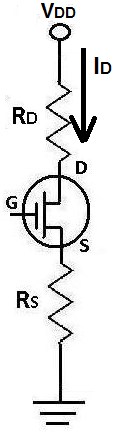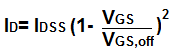﻿ How to Calculate the Drain Current I<small>D</small> of a JFET Transistor?

# How to Calculate the Drain Current ID of a JFET TransistorIf you look at the characteristics curve for the drain current ID of a transistor, it changes drastically during the different regions of the curve.

During the ohmic region, it rises linearly. During the saturation region, it levels off and is much more constant.

Therefore, calculating the drain current for the ohmic region and calculating the drain current for the saturation region requires two completely separate and different formulas.

### How to Calculate the Drain Current ID in the Ohmic Region

The formula to calculate the drain current in the ohmic region is:### How to Calculate the Drain Current ID in the Active Region

The formula to calculate the drain current in the active region is:Note: VGS,off and IDSS are typically the knowns. You can get their values from looking them up in a data table or on the package they came in.